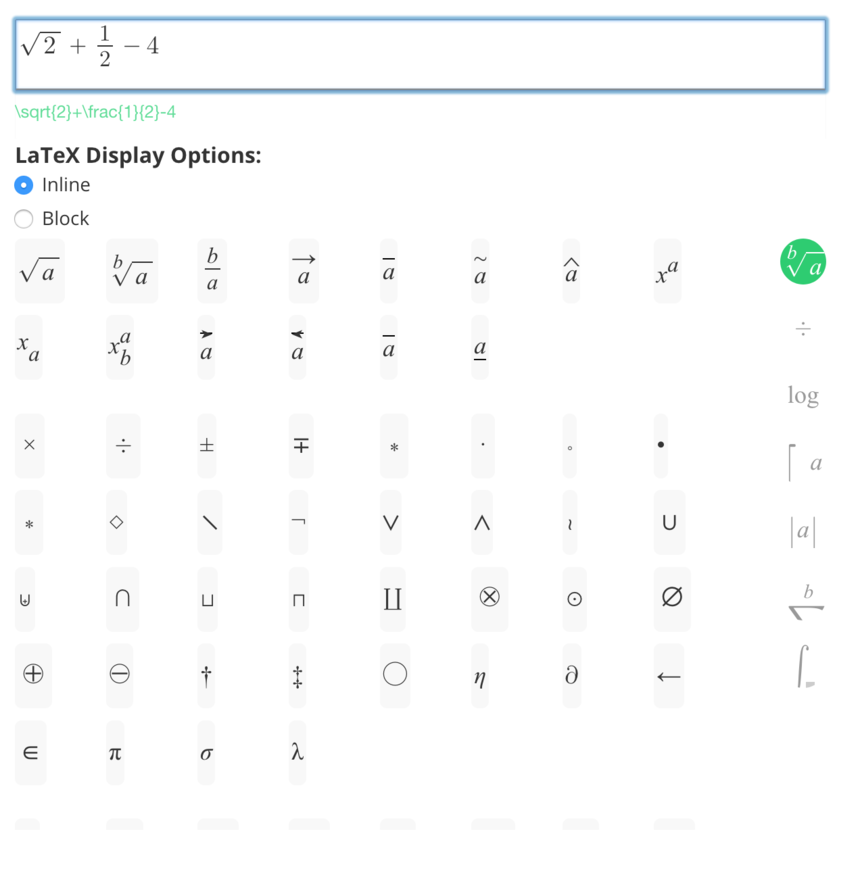# How do I add equations to a page or post?

## Using the "Text" widget

The "Text" widget under the "Content" heading can be dragged on to an OpenLearning page for the authoring of formatted text, embedded into an OpenLearning page. This text editor supports equation editing for page authors.

## Using the "Post Text" widget

The "Post Text" widget under the "Posting" heading can be dragged on to an OpenLearning page for learners to author formatted text, which can then be shared with teachers, class members, or groups. This text editor supports equation editing for learners creating posts.

## Inserting an Equation

These widgets support two methods for adding mathematical equations to text content:

1. Using MathJax syntax:
• $$...$$ for equations that should appear in their own centered content block (e.g. $$\sqrt{2}$$ )
• $$...$$ for equations that appear in-line, within the content (e.g. $$\sqrt{2}$$ )
2. Using the "Equation Editor":
• For the "Text Widget": Select the "Insert" menu in the text editor, and select "Equation". For the "Post Text Widget": Select the "Insert Equation" icon on the top bar.
• The following equation editor will show:• The equation can be edited by either:
• scrolling through symbols and selecting to insert,
• typing in the top input (using "MathQuill" editing), or
• by selecting and editing the MathJax/LaTeX syntax (shown in small green text below the editable equation).
• Once the equation is authored, selecting "Insert Equation" will insert the required MathJax syntax into the page or post.
• N.B. Double-clicking a MathJax equation which has been inserted using the Equation Editor will open the Equation Editor again for further editing.

Upon saving and viewing the page, or upon previewing the post, the MathJax syntax will be displayed (rendered as the full equation).